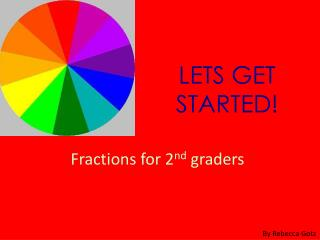DownloadDownload PresentationLETS GET STARTED!

# LETS GET STARTED!

Télécharger la présentation## LETS GET STARTED!

- - - - - - - - - - - - - - - - - - - - - - - - - - - E N D - - - - - - - - - - - - - - - - - - - - - - - - - - -
##### Presentation Transcript

1. LETS GET STARTED! Fractions for 2nd graders By Rebecca Gotz

2. What is a fraction? A fraction is a part of a whole. 1 Whole ½ Half 1/3 One Third

3. Fraction Vocab • Numerator: The number that goes on top of the fraction bar. For Example: one-fourth = 1/4 • Denominator: The number that goes below the fraction bar. For example: one-half= 1/2 • Improper fraction: A number with a larger numerator than denominator. • Mixed fraction: A whole paired with a fraction. When an improper is reduced we end up with a mixed number. Four-thirds = 4/3= 1 1/3

4. Common Fractions • One Third: 1/3 • One Fourth: ¼ • One Half: ½ • Two Thirds: 2/3 • Three Fourths: ¾

5. Handout Work with a partner to answer the questions!

6. Greater Or Less Than Using this chart you can see which fractions are greater or less than others. For example is 1/3 greater or less than 4/8? Answer: Less than.

7. Extra Practice • http://www.kidsolr.com/math/fractions.html